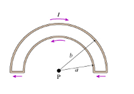# Problem: A loop of wire is in the shape of two concentric semicircles as shown. (Figure 1) The inner circle has radius a; the outer circle has radius b. A current I flows clockwise through the outer wire and counterclockwise through the inner wire. What is the magnitude of the magnetic field at the center of the semicircles?

###### FREE Expert Solution

The magnetic field at the center of the semicircle due to inner wire of radius a is expressed as:

${\mathbit{B}}_{\mathbf{a}}\mathbf{=}\frac{\mathbf{1}}{\mathbf{2}}\frac{{\mathbf{\mu }}_{\mathbf{0}}\mathbf{i}}{\mathbf{2}\mathbf{a}}$

Similarly, the magnetic field at the center of the semicircle due to the outer wire of radius b is:

${\mathbit{B}}_{\mathbf{b}}\mathbf{=}\frac{\mathbf{1}}{\mathbf{2}}\frac{{\mathbf{\mu }}_{\mathbf{0}}\mathbf{i}}{\mathbf{2}\mathbf{b}}$

95% (467 ratings)###### Problem Details

A loop of wire is in the shape of two concentric semicircles as shown. (Figure 1) The inner circle has radius a; the outer circle has radius b. A current I flows clockwise through the outer wire and counterclockwise through the inner wire. What is the magnitude of the magnetic field at the center of the semicircles?